Courses

# Test: Progression (AP And GP)- 3

## 15 Questions MCQ Test Quantitative Aptitude (Quant) | Test: Progression (AP And GP)- 3

Description
This mock test of Test: Progression (AP And GP)- 3 for Quant helps you for every Quant entrance exam. This contains 15 Multiple Choice Questions for Quant Test: Progression (AP And GP)- 3 (mcq) to study with solutions a complete question bank. The solved questions answers in this Test: Progression (AP And GP)- 3 quiz give you a good mix of easy questions and tough questions. Quant students definitely take this Test: Progression (AP And GP)- 3 exercise for a better result in the exam. You can find other Test: Progression (AP And GP)- 3 extra questions, long questions & short questions for Quant on EduRev as well by searching above.
QUESTION: 1

### A group of friends have some money which was in an increasing GP. The total money with the first and the last friend was Rs 66 and the product of the money that the second friend had and that the last but one friend had was Rs 128. If the total money with all of them together was Rs 126, then how many friends were there?

Solution:

The sum of money with the first and the last friend = 66. This can be used as a hint. Let us assume the first friend was having Rs 2 and the last friend was having Rs 64. So, the money can be in the sequence 2, 4, 8, 16, 32, 64. It satisfies the given conditions. Alternatively, this can be done by using the formula for tn of GP also.

QUESTION: 2

### The AM o f two given positive numbers is 2. I f the larger number is increased by 1, the GM of the numbers becomes equal to the AM of the given numbers. Then the HM of the given numbers is

Solution:

Let the numbers be a and b. let a be the larger.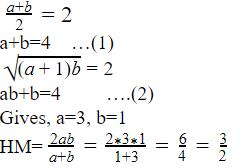QUESTION: 3

### A GP consists of 1000 terms. The sum of the terms occupying the odd places is Pj and the sum of the terms occupying the even places is P2. Find the common ratio of this GP.

Solution:

Take any small sample of 4 terms of any GP viz. 1, 2, 4 and 8. Its common ratio = 2 = (8+2)/(1 +4) And this will be true for any number of terms as long as we are taking total number of terms as even(in this question, it is given as 1000). However, if the total number of terms is given as odd, we cannot find the common ratio in this way, because the number of odd terms ≠ number of even terms.

QUESTION: 4

If the roots of x3 - 12x2 + 39x - 28 = 0 are in an AP then their common difference is

Solution:

Factorize the equation and we get ( x - 1 ) ( x - 4 ) ( x - 7 )

QUESTION: 5

If the nth term of AP is p and the mth term of the same AP is q, then (m + n)th term of AP is

Solution:

Tn = p = a + (n - l ) d

Tm = q = a + (m - l ) d

Add the two and solve through the options given.

QUESTION: 6

If a, b and c are positive integers, then find the product of (a + b) (b + c) (c + a).

Solution:

Put a= l, b = 2 c = 3 and check through options.

QUESTION: 7

The mid-points of the adjacent sides of a square are joined. Again the mid-points of the adjacent sides of the newly formed figure are connected and this process is repeated again and again. Calculate the sum of the areas of all such figures given that the diagonal of outermost square is 6√2cm.

Solution:

The side of the outermost square is 6 cm. Area of the square one in G.P with first term 36 and common ratio = 1/2

QUESTION: 8

There are three numbers in arithmetic progression. If the two larger numbers are both increased by one, then the resulting numbers are prime. The product of these two primes and the smallest of the original numbers is 598. Find the sum of the three numbers.

Solution:
QUESTION: 9

In how many ways can we select three natural numbers out of the first 10 natural numbers so that they are in a geometric progression with the common ratio greater than 1?

Solution:

Make pair of three numbers. Only sequence with 2 and 3 as the common ratio is possible.

QUESTION: 10

If the sum of the first 2n terms of the AP 2, 5, 8... is equal to the sum o f first n terms o f the AP 5 7 ,5 9 , 6 . . . , then what is the value of nl

Solution: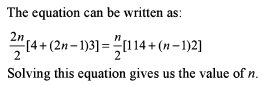QUESTION: 11

Given that (m +1l)th, (n + 1)th and (r +1l)th term of an AP are in GP and m , n , r are in HP, then find the ratio of the first term of the AP to its common difference in terms of n.

Solution:

Since the (m + 1)th, (n + 1)th and (r + 1)th term of an A.P are in G.P. so,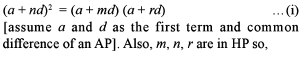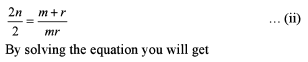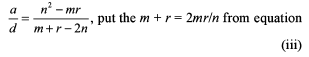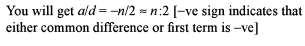QUESTION: 12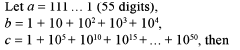Solution: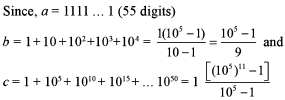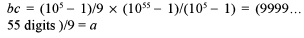QUESTION: 13

If a geometric mean of two non-negative numbers is equal to their harmonic mean, then which of the following is necessarily true?I. One of the numbers is zero.II. Both the numbers are equal.III. One of the numbers is one.

Solution: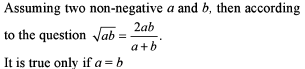QUESTION: 14

A series in which any term is the sum of the preceding two terms is called a Fibonacci series. The first two terms are given initially and together they determine the entire series. If the difference of the squares of the ninth and the eighth terms of a Fibonacci series is 715 then, what is the 12th term of that series?

Solution:

The series is like 1, 1, 2, 3, 5, 8, 13, 21, 34, 55, 89, 144...
The difference is 715 and the 12th term is 144.

QUESTION: 15

The first term of an AP = the common ratio of a GP and the first term of the GP = common difference of the AP. If the sum of the first two terms of the GP is equal to the sum of the first 2 terms of the AP, then the ratio of the first term of the GP to the first term of an AP is

Solution: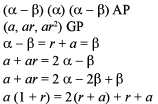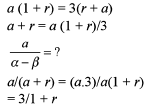This is independent of the first term of GP.